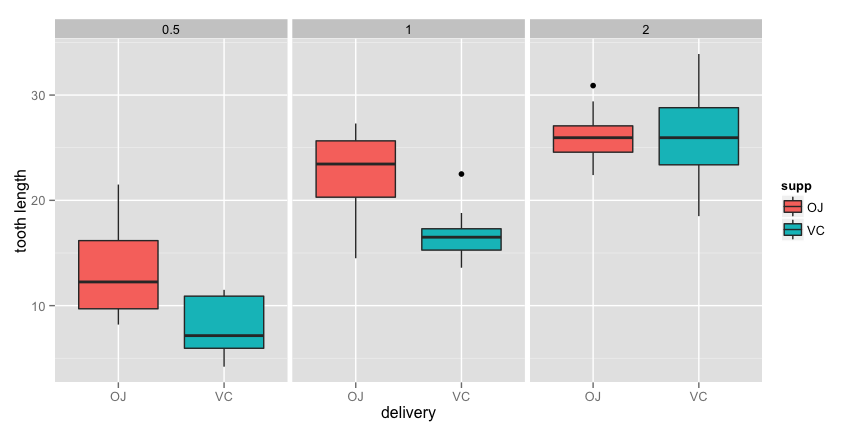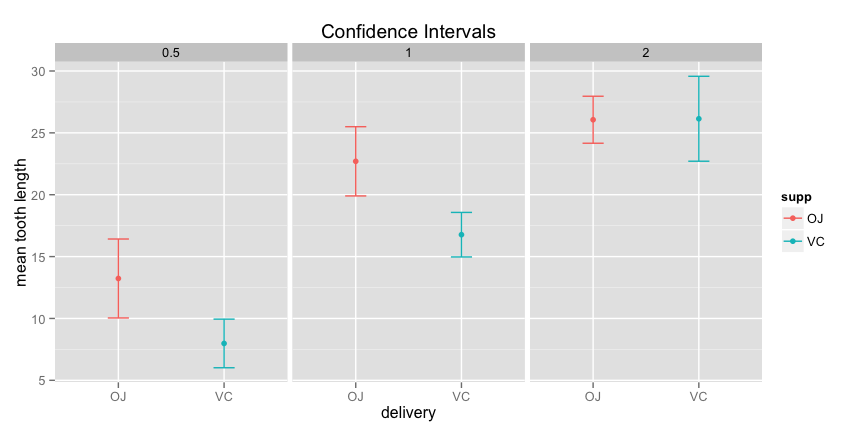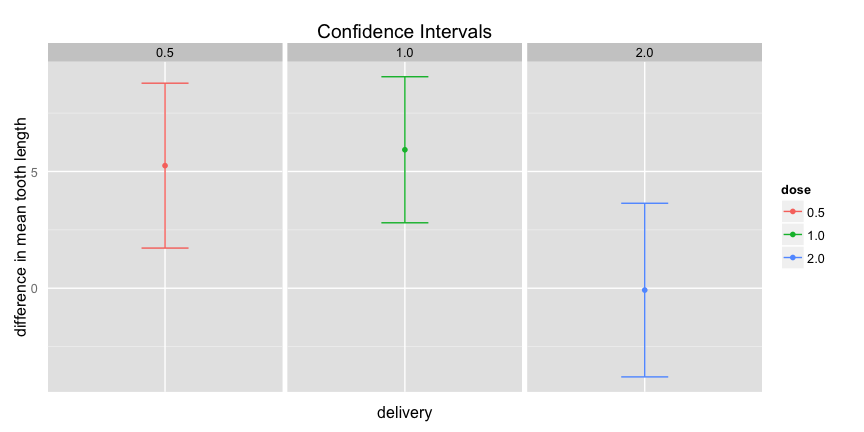The Introduction

From the R manual:

The response is the length of odontoblasts (teeth) in each of 10 guinea pigs at each of three dose levels of Vitamin C (0.5, 1, and 2 mg) with each of two delivery methods (orange juice or ascorbic acid).

We will perform an analysis of this data to see if there are any significant differences in tooth growth for the different delivery methods across dosages.

The Data

Lets take a look at the data:

##    len supp dose
## 1  4.2   VC  0.5
## 2 11.5   VC  0.5
## 3  7.3   VC  0.5
## 4  5.8   VC  0.5
## 5  6.4   VC  0.5
## 6 10.0   VC  0.5

and now lets take a look at the means of the data, grouped by dose and supp:

aggregate(len ~ dose + supp, ToothGrowth, mean);
##   dose supp   len
## 1  0.5   OJ 13.23
## 2    1   OJ 22.70
## 3    2   OJ 26.06
## 4  0.5   VC  7.98
## 5    1   VC 16.77
## 6    2   VC 26.14

We can see, within dosages, and with the exception of 2.0 dosage, that the means vary. Lets illustrate this with some visualizations:

plot <- ggplot(ToothGrowth, aes(x = supp, y = len, fill = supp));
plot <- plot + geom_boxplot();
plot <- plot + facet_grid(~ dose);
plot <- plot + xlab("delivery");
plot <- plot + ylab("tooth length");
plot;from looking at the boxplots it’s clear that dosage 0.5 and 1.0 have different medians for the different deliveries. On the other hand, there doesn’t seem to be a difference between the medians of the deliveries for dosage 2.0. Lets take a look at the confidence intervals for the different means across dosage and delivery:

conf.int <- function(x) {
n    = length(x);
mean = mean(x);
sd   = sd(x);
se   = sd / sqrt(n);
t    = qt(0.975, df = n - 1);
c(mean = mean, ci_lower = mean - t * se, ci_upper = mean + t * se);
}

ci        <- do.call(data.frame, aggregate(len ~ dose + supp, ToothGrowth, conf.int));
names(ci) <- c('dose', 'supp', 'mean', 'ci_lower', 'ci_upper');

plot <- ggplot(ci, aes(x = supp, y = mean, colour = supp));
plot <- plot + geom_point();
plot <- plot + geom_errorbar(aes(ymin = ci_lower, ymax = ci_upper), width = 0.2);
plot <- plot + facet_grid(~ dose);
plot <- plot + ggtitle("Confidence Intervals");
plot <- plot + xlab("delivery");
plot <- plot + ylab("mean tooth length");
plot;this shows that the deliveries of both dosage 0.5 and 1.0 have different means - even their confidence intervals don’t overlap - but the means of the deliveries for dosage 2.0 appear to be the same.

The Analysis

Lets run some hypothesis tests across the different dosages to see if the means are significantly different.

we assume the samples are independent, and that the subjects are randomly assigned. Also, as the size of each sample is less than 10% of the size of the population, we have met the necessary conditions to perform our hypothesis tests. First we prepare our data:

dose_0.5    <- subset(ToothGrowth, dose == 0.5);
dose_1.0    <- subset(ToothGrowth, dose == 1.0);
dose_2.0    <- subset(ToothGrowth, dose == 2.0);

dose_0.5_VC <- subset(dose_0.5, supp == 'VC');
dose_1.0_VC <- subset(dose_1.0, supp == 'VC');
dose_2.0_VC <- subset(dose_2.0, supp == 'VC');

dose_0.5_OJ <- subset(dose_0.5, supp == 'OJ');
dose_1.0_OJ <- subset(dose_1.0, supp == 'OJ');
dose_2.0_OJ <- subset(dose_2.0, supp == 'OJ');

then we run our tests. We note that the data isn’t paired. Also from looking at the boxplots it looks as if the samples have different variances - in fact, when in doubt, we should err on the side of unequal variances.

dose_0.5_test <- t.test(dose_0.5_OJ$len, dose_0.5_VC$len);
dose_1.0_test <- t.test(dose_1.0_OJ$len, dose_1.0_VC$len);
dose_2.0_test <- t.test(dose_2.0_OJ$len, dose_2.0_VC$len);

the first test looks at the difference between the two delivery methods across the 0.5 dosage:

dose_0.5_test;
##
##  Welch Two Sample t-test
##
## data:  dose_0.5_OJ$len and dose_0.5_VC$len
## t = 3.1697, df = 14.969, p-value = 0.006359
## alternative hypothesis: true difference in means is not equal to 0
## 95 percent confidence interval:
##  1.719057 8.780943
## sample estimates:
## mean of x mean of y
##     13.23      7.98

with a p-value of 0.0063586 we reject the null hypothesis that the true difference in means is equal to zero at the 5% significance level. Looking at the associated confidence interval (1.7190573, 8.7809427) we see it doesn’t include 0, which is what we would expect at that p-value. The next test looks at the difference between the two delivery methods across the 1.0 dosage:

dose_1.0_test;
##
##  Welch Two Sample t-test
##
## data:  dose_1.0_OJ$len and dose_1.0_VC$len
## t = 4.0328, df = 15.358, p-value = 0.001038
## alternative hypothesis: true difference in means is not equal to 0
## 95 percent confidence interval:
##  2.802148 9.057852
## sample estimates:
## mean of x mean of y
##     22.70     16.77

with a p-value of 0.0010384 we reject the null hypothesis that the true difference in means is equal to zero at the 5% significance level. Looking at the associated confidence interval (2.8021482, 9.0578518) we see it doesn’t include 0, which is what we would expect at that p-value. The next test looks at the difference between the two delivery methods across the 2.0 dosage:

dose_2.0_test;
##
##  Welch Two Sample t-test
##
## data:  dose_2.0_OJ$len and dose_2.0_VC$len
## t = -0.0461, df = 14.04, p-value = 0.9639
## alternative hypothesis: true difference in means is not equal to 0
## 95 percent confidence interval:
##  -3.79807  3.63807
## sample estimates:
## mean of x mean of y
##     26.06     26.14

with a p-value of 0.9638516 we fail to reject the null hypothesis that the true difference in means is equal to zero at the 5% significance level. Looking at the associated confidence interval (-3.7980705, 3.6380705) we see it includes 0, which is what we would expect at that p-value. Lets visualize the confidence intervals:

dose_0.5_CI <- dose_0.5_test$conf.int; dose_1.0_CI <- dose_1.0_test$conf.int;
dose_2.0_CI <- dose_2.0_test$conf.int; dose_0.5_M <- dose_0.5_test$estimate - dose_0.5_test$estimate; dose_1.0_M <- dose_1.0_test$estimate - dose_1.0_test$estimate; dose_2.0_M <- dose_2.0_test$estimate - dose_2.0_test\$estimate;

stats <- data.frame(
supp       = factor(c('0', '0', '0')),
dose       = factor(c('0.5', '1.0', '2.0')),
difference = c(dose_0.5_M, dose_1.0_M, dose_2.0_M),
ci_lower   = c(dose_0.5_CI, dose_1.0_CI, dose_2.0_CI),
ci_upper   = c(dose_0.5_CI, dose_1.0_CI, dose_2.0_CI)
);

plot <- ggplot(stats, aes(x = supp, y = difference, colour = dose));
plot <- plot + geom_point();
plot <- plot + geom_errorbar(aes(ymin = ci_lower, ymax = ci_upper), width = 0.2);
plot <- plot + facet_grid(~ dose);
plot <- plot + theme(axis.ticks = element_blank(), axis.text.x = element_blank());
plot <- plot + guides(fill = FALSE);
plot <- plot + ggtitle("Confidence Intervals");
plot <- plot + xlab("delivery");
plot <- plot + ylab("difference in mean tooth length");
plot;The Conclusion

It seems clear that for the 0.5 and 1.0 dosages there is a significant difference between the different deliveries and the effects on tooth growth, with orange juice being superior to ascorbic acid. There is no significant difference between the deliveries for the 2.0 dosage.